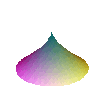### Singularities

BackA1-- x^2-y^2-z^2 A1++ A isolated point x^2+y^2+z^2A2-- x^3-y^2-z^2A2+- x^3+y^2-z^2A3-- x^4-y^2-z^2A3+- x^4+y^2-z^2A4-- x^5-y^2-z^2A4+- x^5+y^2-z^2D4-- x^2 y-y^3-z^2D4+- x^2 y+y^3-z^2D5-- x^2 y-y^4-z^2D5+- x^2 y+y^4-z^2D6-- x^2 y-y^5-z^2D6+- x^2 y+y^5-z^2E6-- x^3-y^4-z^2E6+- x^3+y^4-z^2E7 x^3-x y^3-z^2E8 x^3-y^5-z^2 The above is the complete list of 'simple' singularities. There are many more which are not simple, such as:T[3,3,3] x^3+y^3+z^3+2 x y z Not all singularities are isolated. Some surfaces can contain a whole line of singular points such as:Whitney Umberella (cross cap) x^2 z-y^2Swallowtail